# Standard deviation calculator

Enter sample or population data in the given input box to find standard deviation using standard deviation calculator.

Give Us Feedback

## Standard deviation calculator

Standard deviation calculator is used to find the standard deviation, variance, mean, and the statistical sum of squares. This deviation calculator takes a sample and population set of data values.

## How to use a standard deviation calculator?

Here are a few steps to use the above std calculator.

Step 1: Choose the type of data set.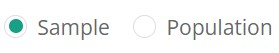Step 2: Enter comma-separated sample or population data values into the required box.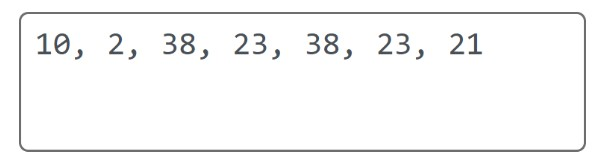Step 3: Hit the calculate button to get the result.Step 4: The result will show below the calculate button.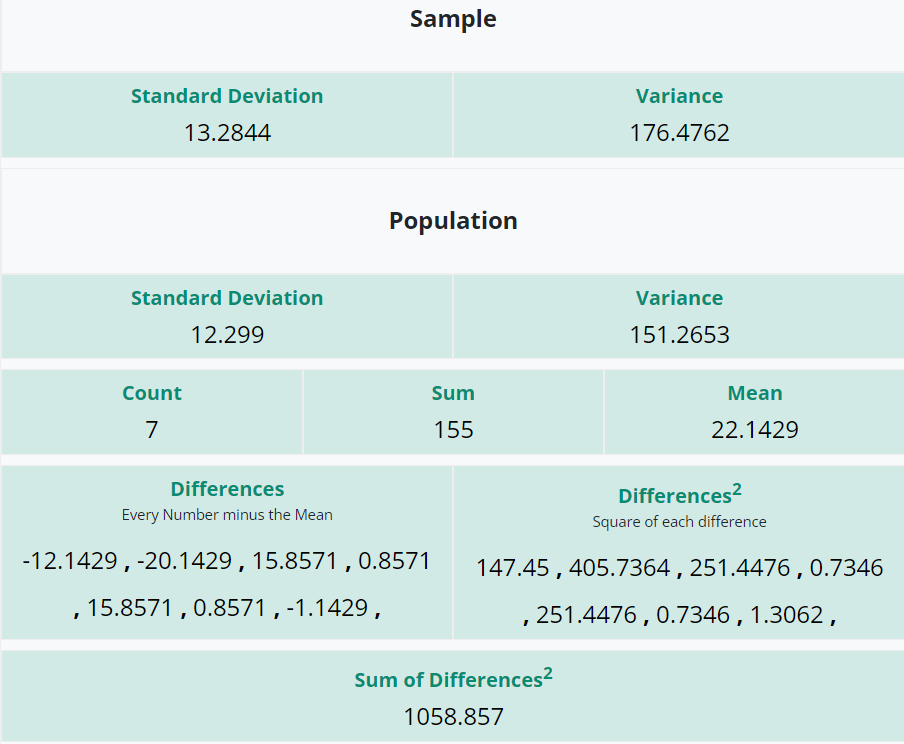## What is the standard deviation?

The measure of the spread of data distribution is known as standard deviation. It measures the distance between each data observation and the mean. The standard deviation is of two types:

• Sample standard deviation
• Population standard deviation

If the given data is the population of its own, then divide the sum of squares by N.
If the given data is a sample from a larger population, then the sum of squares must be divided by n – 1.

## Standard deviation formula

The formula for the population standard deviation is: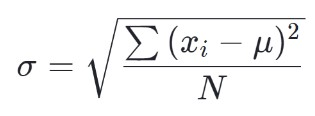The formula for the sample standard deviation is: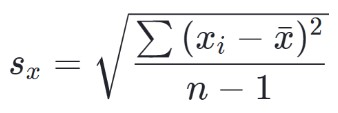## How to calculate standard deviation?

Below are a few solved examples of standard deviation.

Example 1: For population standard deviation

Find the population standard deviation of 8, 22, 26, 25, 30, and 33.

Solution

Step I: Find the mean of the given population data.

Mean of population data = Σx/n
= [8 + 22 + 26 + 25 + 30 + 33]/6
= 144/6
= 24

Step II: Now find the typical distance of each data point & the mean and square of each deviation.

 Data values (xi) xi - µ (xi - µ)2 8 8 – 24 = -16 (-16)2 = 256 22 22 – 24 = -2 (-2)2 = 4 26 26 – 24 = 2 (2)2 = 4 25 25 – 24 = 1 (1)2 = 1 30 30 – 24 = 6 (6)2 = 36 33 33 – 24= 9 (9)2 = 81

Step III: Add the deviations to find the statistical sum of squares.

Σ(xi - µ)2 = 256 + 4 + 4 + 1 + 36 + 81

Σ(xi - µ)2 =  382

Step IV: Now divide the sum of squares by n.

Σ(xi - µ)2/n = 382/6

Σ(xi - µ)2/n = 63.667

Step V: Take the square root.

√[Σ(xi - µ)2/n] = √63.667

√[Σ(xi - µ)2/n] = 7.979

Use the population standard deviation calculator above to solve this problem quickly.

Example 2: For sample standard deviation

Find the sample standard deviation of 12, 15, 18, 20, 25.

Solution

Step I: Find the mean of the given sample data.

Mean of sample data = Σx/n
= [12 + 15 + 18 + 20 + 25]/5
= 90/5
= 18

Step II: Now find the typical distance of each data point & the mean and square of each deviation.

 Data values (xi) xi - x̅ (xi - x̅)2 12 12 – 18 = -6 (-6)2 = 36 15 15 – 18 = -3 (-3)2 = 9 18 18 – 18 = 0 (0)2 = 0 20 20 – 18 = 2 (2)2 = 4 25 25 – 18 = 7 (7)2 = 49

Step III: Add the deviations to find the statistical sum of squares.

Σ(xi - x̅)2 = 36 + 9 + 0 + 4 + 49

Σ(xi - x̅)2 =  98

Step IV: Now divide the sum of squares by n – 1.

Σ(xi - x̅)2 / n-1 = 98/5-1

Σ(xi - x̅)2 / n-1 = 98/4

Σ(xi - x̅)2 / n-1 = 24.5

Step V: Take the square root.

√[Σ(xi - x̅)2 /n-1] = √24.5

√[Σ(xi - x̅)2 /n-1] = 4.95

### Math Tools